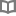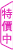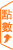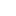人生很難，但可以用自信的台步踢破鐵板 - 金禧 - BOOKWALKER中文電子書

人生很難，但可以用自信的台步踢破鐵板

金禧

台灣最早闖蕩國際伸展台的名模之一，2006年隻身前往巴黎，從國際伸展台上紅回台灣，入行超過16年，目前已是「資深前輩」等級，憑藉個人特色強烈的丹鳳眼、對時尚的敏銳度以及同為模特兒的法國老公支持，雖然已是育有一子一女的法式辣媽，在時尚界仍然擁有持續不墜的影響力。

• 放入購物車
•試閱

• 放入購物車
•試閱

• 放入購物車
•試閱• 放入購物車
•試閱• 放入購物車
•試閱• 放入購物車
•試閱

• 放入購物車
•試閱

• 放入購物車
•試閱

• 放入購物車
•試閱

• 放入購物車
•試閱

• 放入購物車
•試閱

• 放入購物車
•試閱

• 放入購物車
•試閱

• 放入購物車
•試閱

• 放入購物車
•試閱

• 放入購物車
•試閱

• 放入購物車
•試閱• 放入購物車
•試閱

• 放入購物車
•試閱

• 放入購物車
•試閱

• 放入購物車
•試閱• 放入購物車
•試閱

• 放入購物車
•無試閱

• 放入購物車
•試閱

• 放入購物車
•試閱

• 放入購物車
•試閱

• 放入購物車
•試閱

• 放入購物車
•試閱

• 放入購物車
•試閱

• 放入購物車
•試閱

• 放入購物車
•試閱• 放入購物車
•試閱• 放入購物車
•試閱

• 放入購物車
•試閱

• 放入購物車
•試閱

• 放入購物車
•試閱

• 放入購物車
•試閱• 放入購物車
•試閱

• 放入購物車
•試閱

• 放入購物車
•試閱

• 放入購物車
•試閱

• 放入購物車
•試閱

• 放入購物車
•試閱